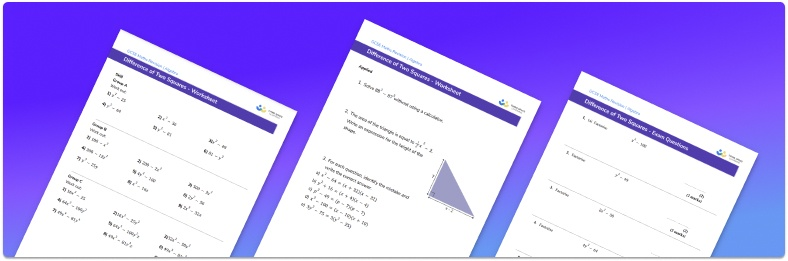# Difference Of Two Squares Worksheet• Section 1 of the difference of two squares worksheet contains 20+ skills-based difference of two squares questions, in 3 groups to support differentiation
• Section 2 contains 3 applied difference of two squares questions with a mix of worded problems and deeper problem solving questions
• Section 3 contains 3 foundation and higher level GCSE exam style questions
• Answers and a mark scheme for all questions are provided
• Questions follow variation theory with plenty of opportunities for students to work independently at their own level
• All questions created by fully qualified expert secondary maths teachers
• Suitable for GCSE maths revision for AQA, OCR and Edexcel exam boards

• This field is for validation purposes and should be left unchanged.

You can unsubscribe at any time (each email we send will contain an easy way to unsubscribe). To find out more about how we use your data, see our privacy policy.

### Difference of two squares at a glance

When a binomial quadratic expression involves a square term subtracting another square term we can factorise it by using the difference of two squares method. This involves two perfect squares being subtracted from each other.

In order to use the difference of two square method, the square roots of both terms need to be found. These terms are then placed into a pair of double brackets with a plus sign in one and a minus sign in the other. This results in the algebraic expression being written as a product of two factors. Knowledge of square numbers, square roots and indices is useful when using this method.

Sometimes, before the difference of two squares method can be used, the expression will need to be factorised into a single bracket by finding a common factor of each of the coefficients and/or constants in the expression.

Factorising using the difference of two squares can be useful when solving quadratic equations or when simplifying algebraic fractions.

Looking forward, students can progress with more factorising worksheets to additional algebra worksheets, for example an expanding brackets worksheet or simultaneous equations worksheet.For more teaching and learning support on Algebra our GCSE maths lessons provide step by step support for all GCSE maths concepts.

## Do you have KS4 students who need more focused attention to succeed at GCSE?There will be students in your class who require individual attention to help them succeed in their maths GCSEs. In a class of 30, it’s not always easy to provide.

Help your students feel confident with exam-style questions and the strategies they’ll need to answer them correctly with our dedicated GCSE maths revision programme.

Lessons are selected to provide support where each student needs it most, and specially-trained GCSE maths tutors adapt the pitch and pace of each lesson. This ensures a personalised revision programme that raises grades and boosts confidence.

Find out more